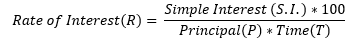# SBI PO: How to Approach SBI PO Simple Interest Problems?

SBI PO is one of the most awaited examinations in the country. Hence, the competition is intense and even the fraction of a mark can make all the difference between selection and rejection of an aspirant’s candidature. Therefore, at Oliveboard, we focus on guiding our students at the most specific level possible, which is by the topic. Simple interest is a topic that is relatively easy and appears in the Quantitative Aptitude section of SBI PO examinations thus making it one of the good ways to earn some marks and boost the overall chances of being selected at SBI. Therefore, to help you do the same, we have brought an article, “SBI PO: How to Approach SBI PO Simple Interest Problems?”

Register Now & Get Free Daily Updates on Upcoming Exams & Courses

## SBI PO: How To Approach SBI PO Simple Interest Problems? – Steps To Approach

SBI PO Simple interest problems are relatively simple and hence they can be attempted easily if the correct method is known for approaching them. To solve SBI PO simple interest problems efficiently, follow the given steps –

• Identify what does the question requires from you? There are only 4 variables in a typical S.I. question including the Simple Interest (S.I.), Time (T), Rate of Interest Per Annum (R%), and Principal (P). You will be provided with any 3 variables and you need to use them into a formula to figure the last one yourself.
• Before you do anything, make note of the following formulas; each of them can be used to figure out what’s not given in the question with whatever information has been provided: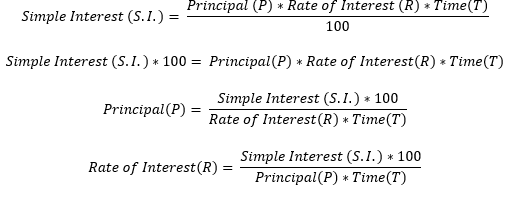Now, let us look at 4 cases where each of those formulas can be applied directly:

• Case 1: What is the simple interest that would accrue on a principal ‘P’ lent at an interest rate of ‘R’% per annum for a time of ‘T’ years? Since in this case all the 3 variables are provided, we only need to put in the basic formula to compute the S.I. and hence: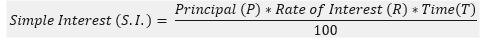• Case 2: When lent at an interest rate ‘R’% per annum for ‘T’ years the accrued simple interest is ‘S.I.’ on a certain amount of money. What’s the amount of money? In this case, the principal needs to be computed but all the other 3 variables have been provided. So, the formula we use would be: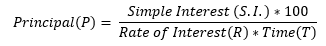• Case 3: When lent at an interest rate of ‘R’% per annum for a specific period, a principal ‘P’ accrues a simple interest of ‘S.I.’. What’s the number of years? This is another case wherein the details have all been provided by the number of years that have not been specified, and hence we need to compute the same. The formula we use to compute the same is: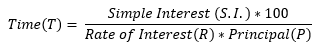• Case 4: When a principal ‘P’ is lent at some interest rate for ‘T’ years, it accrues a Simple Interest ‘S.I.’. What is the annual interest rate? The last case is where all the details have been provided except for that of the rate of interest which is, therefore, the variable to be computed using the formula: